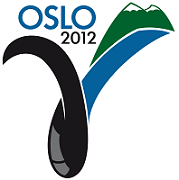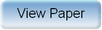Ninth International Geostatistics Congress, Oslo, Norway
June 11 – 15, 2012Session: Plenary 4 Abstract No.: O-008 Title: History matching under uncertain geological scenario Author(s): H. Park, Stanford University (US)J. Caers, Stanford University (US) Abstract: The main interest in this paper lies in obtaining multiple history matched models when the geological scenario for spatial continuity (a discrete variable) is uncertain. Within a Bayesian framework, the only exact sampling techniques for obtaining multiple such Earth models are the well-known Markov chain Monte Carlo samplers such as the rejection or Metropolis sampler. Such samplers are however extremely inefficient for practical applications. To handle uncertainty in geological scenarios within a Bayesian framework for real world situations, we propose to split the problem in two parts: the first part is the traditional question of history matching constrained to a specific prior geologic scenario, hard data, and nonlinear time-dependent data (soft data) for a given geological scenario, the second part is to determine the probability of each geologically possible scenario given the production data. The first problem can be solved efficiently by the post-image, pre-image formulation with a given geological scenario (e.g. a fixed variogram or training image). (see Scheidt et al., this conference). To solve the latter question, we propose an adaptive kernel smoothing technique in metric space. The proposed technique only needs an order of magnitude of 100 prior models and given production data (oil/water production rate, cumulative oil/water production and etc.) to estimate the probability of each geologically possible scenario from these prior models. The estimated probability for each scenario is then used to weigh history matched models for future performance prediction. Comparison with the rejection sampler shows that the proposed technique is accurate at a fraction of the cost of rejection sampling without the need to generate a large amount of history matched models.Produced by X-CD Technologies Inc.